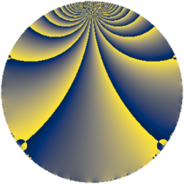# Properties

 Label 182.2.mLevel $182$ Weight $2$ Character orbit 182.m Rep. character $\chi_{182}(43,\cdot)$ Character field $\Q(\zeta_{6})$ Dimension $16$ Newform subspaces $2$ Sturm bound $56$ Trace bound $1$

# Related objects

## Defining parameters

 Level: $$N$$ $$=$$ $$182 = 2 \cdot 7 \cdot 13$$ Weight: $$k$$ $$=$$ $$2$$ Character orbit: $$[\chi]$$ $$=$$ 182.m (of order $$6$$ and degree $$2$$) Character conductor: $$\operatorname{cond}(\chi)$$ $$=$$ $$13$$ Character field: $$\Q(\zeta_{6})$$ Newform subspaces: $$2$$ Sturm bound: $$56$$ Trace bound: $$1$$ Distinguishing $$T_p$$: $$3$$

## Dimensions

The following table gives the dimensions of various subspaces of $$M_{2}(182, [\chi])$$.

Total New Old
Modular forms 64 16 48
Cusp forms 48 16 32
Eisenstein series 16 0 16

## Trace form

 $$16 q + 8 q^{4} - 8 q^{9} + O(q^{10})$$ $$16 q + 8 q^{4} - 8 q^{9} - 4 q^{10} - 12 q^{11} - 8 q^{13} + 8 q^{14} - 12 q^{15} - 8 q^{16} - 4 q^{17} - 4 q^{22} - 8 q^{25} + 24 q^{27} - 4 q^{29} + 16 q^{30} + 24 q^{33} + 8 q^{36} + 12 q^{37} + 16 q^{38} - 48 q^{39} - 8 q^{40} - 36 q^{41} - 4 q^{42} + 12 q^{43} + 60 q^{45} - 12 q^{46} + 8 q^{49} - 12 q^{50} - 40 q^{51} - 4 q^{52} + 56 q^{53} - 36 q^{54} - 4 q^{55} + 4 q^{56} + 24 q^{58} - 8 q^{61} + 12 q^{62} - 12 q^{63} - 16 q^{64} - 24 q^{65} - 16 q^{66} - 12 q^{67} + 4 q^{68} + 20 q^{69} - 12 q^{72} - 40 q^{75} - 4 q^{78} + 8 q^{79} - 36 q^{81} + 8 q^{82} + 12 q^{84} + 60 q^{85} - 4 q^{87} + 4 q^{88} + 16 q^{90} - 20 q^{91} + 48 q^{93} + 16 q^{94} + 36 q^{95} + 48 q^{97} + O(q^{100})$$

## Decomposition of $$S_{2}^{\mathrm{new}}(182, [\chi])$$ into newform subspaces

Label Dim $A$ Field CM Traces $q$-expansion
$a_{2}$ $a_{3}$ $a_{5}$ $a_{7}$
182.2.m.a $4$ $1.453$ $$\Q(\zeta_{12})$$ None $$0$$ $$2$$ $$0$$ $$0$$ $$q+\zeta_{12}q^{2}+(1-\zeta_{12}-\zeta_{12}^{2}+2\zeta_{12}^{3})q^{3}+\cdots$$
182.2.m.b $12$ $1.453$ $$\mathbb{Q}[x]/(x^{12} - \cdots)$$ None $$0$$ $$-2$$ $$0$$ $$0$$ $$q-\beta _{5}q^{2}+(\beta _{2}+\beta _{10})q^{3}+(1-\beta _{7})q^{4}+\cdots$$

## Decomposition of $$S_{2}^{\mathrm{old}}(182, [\chi])$$ into lower level spaces

$$S_{2}^{\mathrm{old}}(182, [\chi]) \cong$$ $$S_{2}^{\mathrm{new}}(13, [\chi])$$$$^{\oplus 4}$$$$\oplus$$$$S_{2}^{\mathrm{new}}(91, [\chi])$$$$^{\oplus 2}$$